Function Repository Resource:

# BinormalSurface

Compute the binormal surface to a curve

Contributed by: Alfred Gray
 ResourceFunction["BinormalSurface"][c,t,{u,v}] computes the binormal surface of a curve c with parameter t using u and v to parametrize the result.

## Details and Options

The binormal surface associated to a curve is generated by its binormal vector field.
The binormal surface is effectively a ruled surface.

## Examples

### Basic Examples (4)

Define and plot a helix curve:

 In:=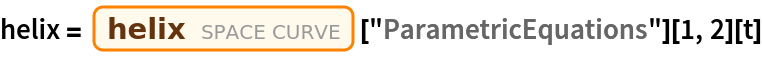Out=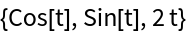In:=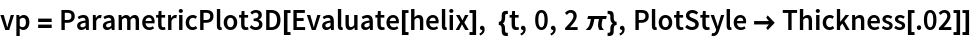Out=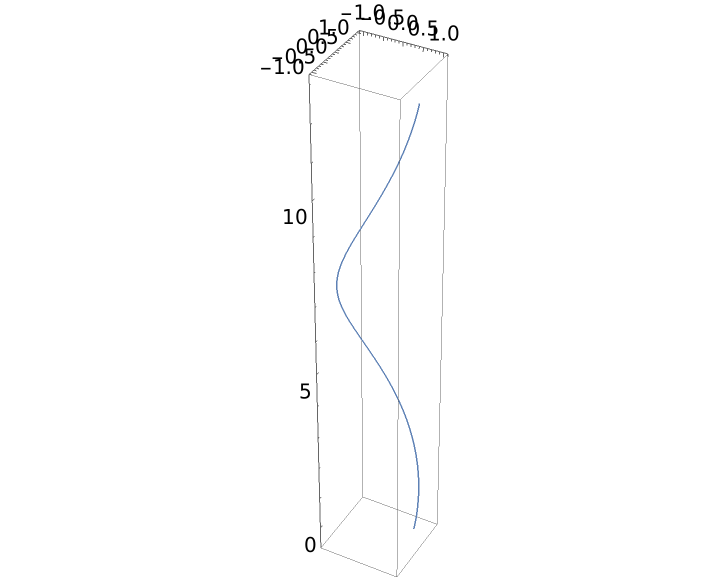Compute the normal surface of the helix curve with the resource function NormalSurface and plot the result:

 In:=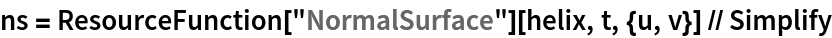Out=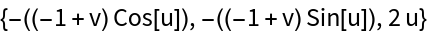In:=Out=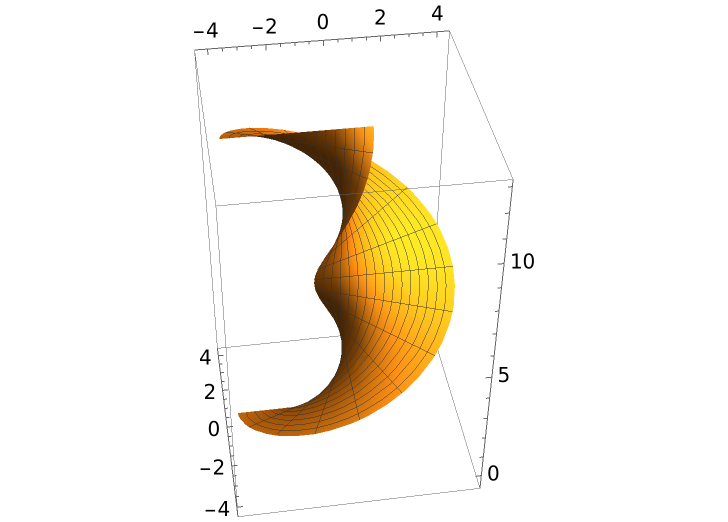Compute and plot the binormal surface of the helix curve:

 In:=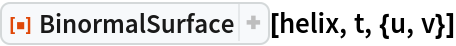Out=In:=Out=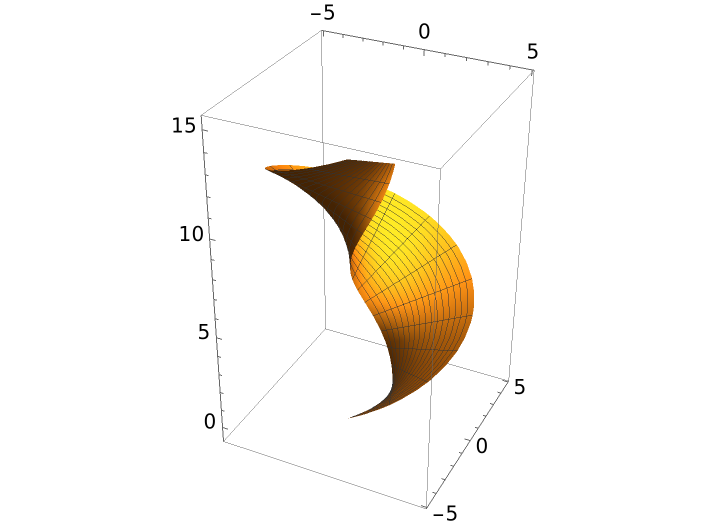Show both surfaces along with the helix curve:

 In:=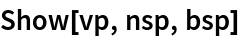Out=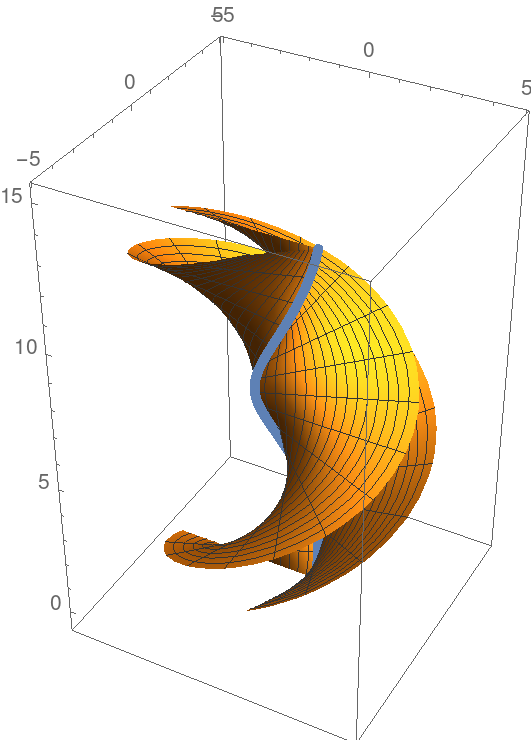Enrique Zeleny

## Version History

• 1.0.0 – 24 February 2020